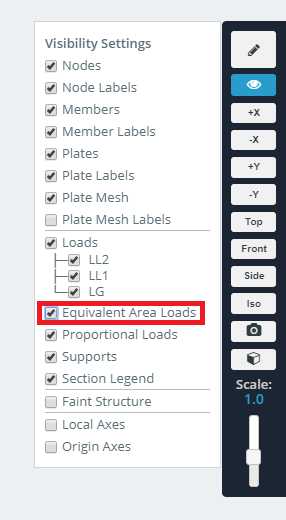Documentation [Deprecated]
SkyCiv Documentation

Getting Started

Section Builder
Operations
Built-Up and Composite Sections
Custom Shapes

Member Design
General
Member Design Modules
Code Verification

RC Design
General
Code Verification

Area loads are equivalent distributed loads (DL's) that are applied to members. They are generated from the consideration of a pressure load (that would be applied to a plate) and how the plate (such as a slab) would transmit its load to the structural members which support it. Area loads are useful when not wanting to model the plate, which may introduce unwanted stiffness into the model. Like with other loads, you can assign a load group to area loads.

SkyCiv offers 3 types of area loads:

• One-way (open):
• Takes in 2 members
• Creates quadrilateral distributions to the two members
• One-way (closed):
• Takes in 3 or 4 nodes
• Creates rectangular distributions to the members between the nodes.
• Two-way:
• Takes in 3 or 4 nodes.
• For 3 nodes it creates triangular distributions
• For 4 nodes it creates a pair of triangular distributions and a pair of quadrilateral distributions
• Applies variable pressures to columns via distributed loads
• Takes in 3 or 4 nodes (the face of where the pressure will be applied)
• Elevations of where the wind loads change
• The pressures for each range of elevations

The following examples will go through each type of area load and their differences.

# Example: One Way (Open) Area Load

In this example we’ll be looking at how one way area loads can be applied to a frame which supports a slab. It is assumed that the slab is being supported directly by the beams 7-10. As such, the system is considered one-way, and no distributed load needs to be applied to the girders 1-6. The slab sits on top of this frame, from edge to edge with a pressure load of 1.2 ksf. The area load will create distributed loads (DL’s) with a rectangular distribution.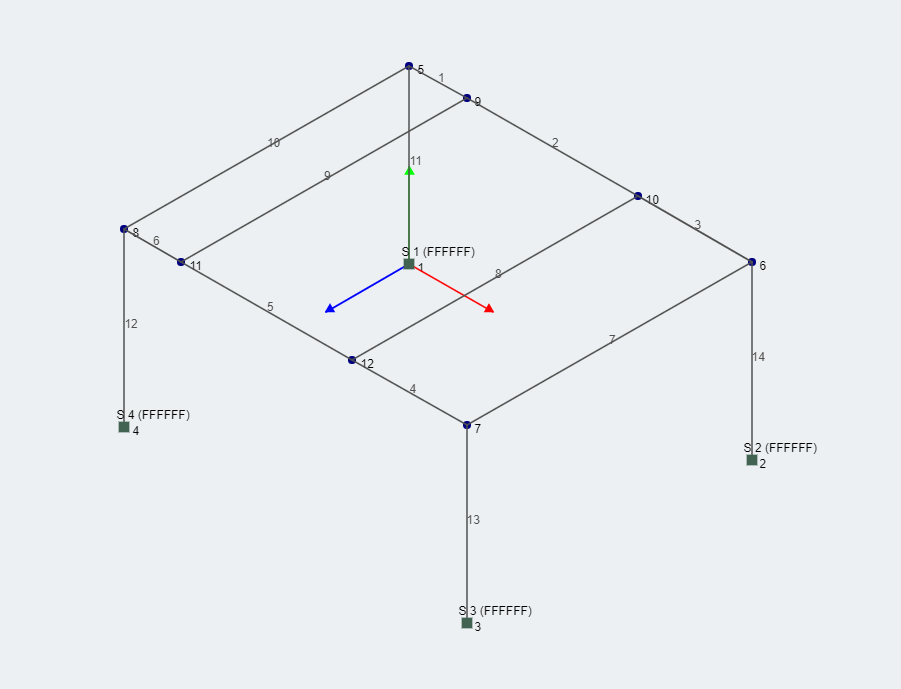To do this in SkyCiv, click the “Area Loads” button to open the menu. Select “One Way (Open Area)”. Then enter in two member ID’s. The name “open area” refers to the fact that the two members themselves do not form a closed polygon, and DL’s only form on these two members. Do this for members 7-8, 8-9, and 9-10. Note also the fact that there are two DL's on members 8 and 9. This is because these members lie in two areas that were selected to form area loads.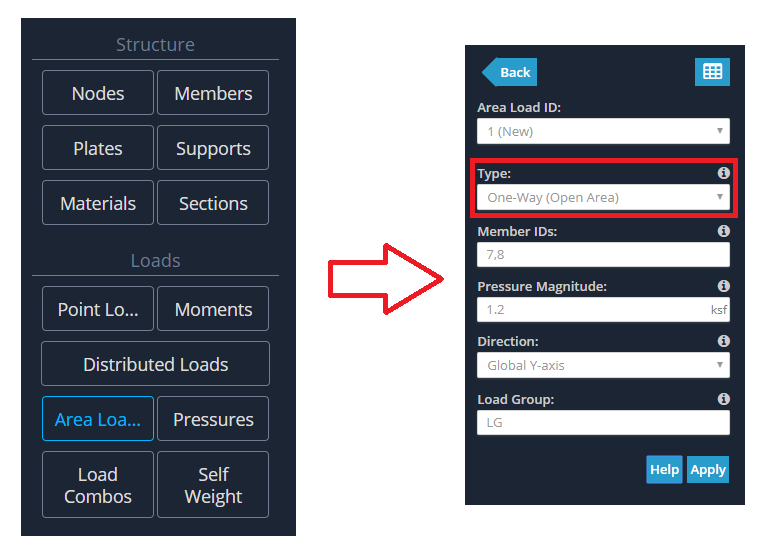By default, the area load will be represented by a pressure load and magnitude as shown below.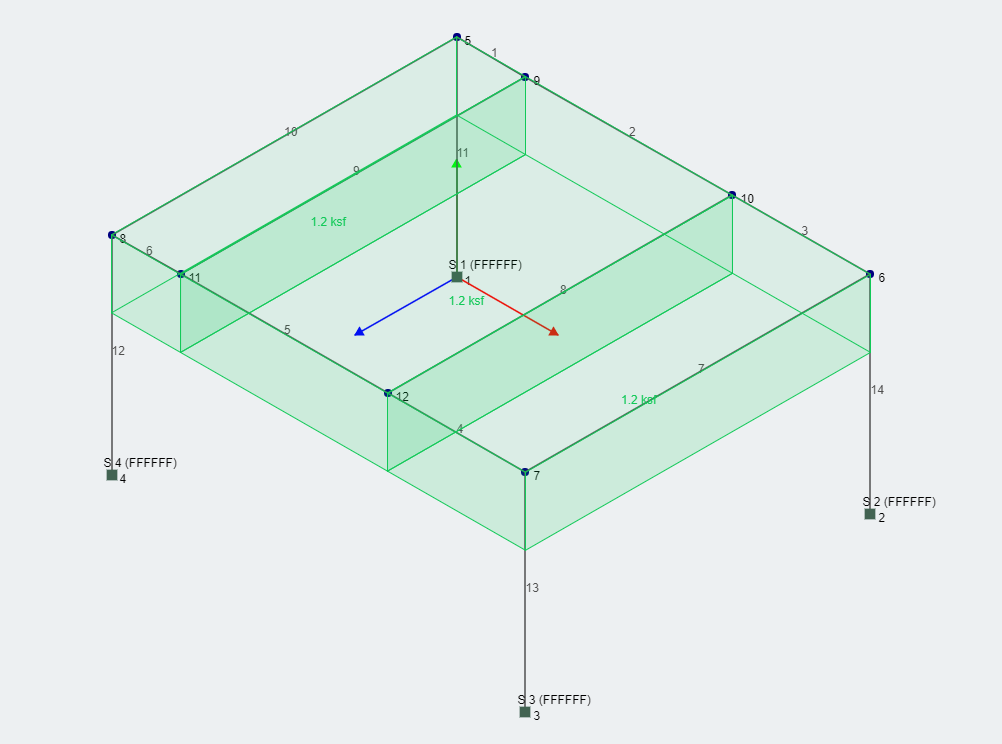You are able to toggle the view from pressure view to equivalent area load (DL) form by clicking the view icon, and checking the box "Equivalent Area Loads".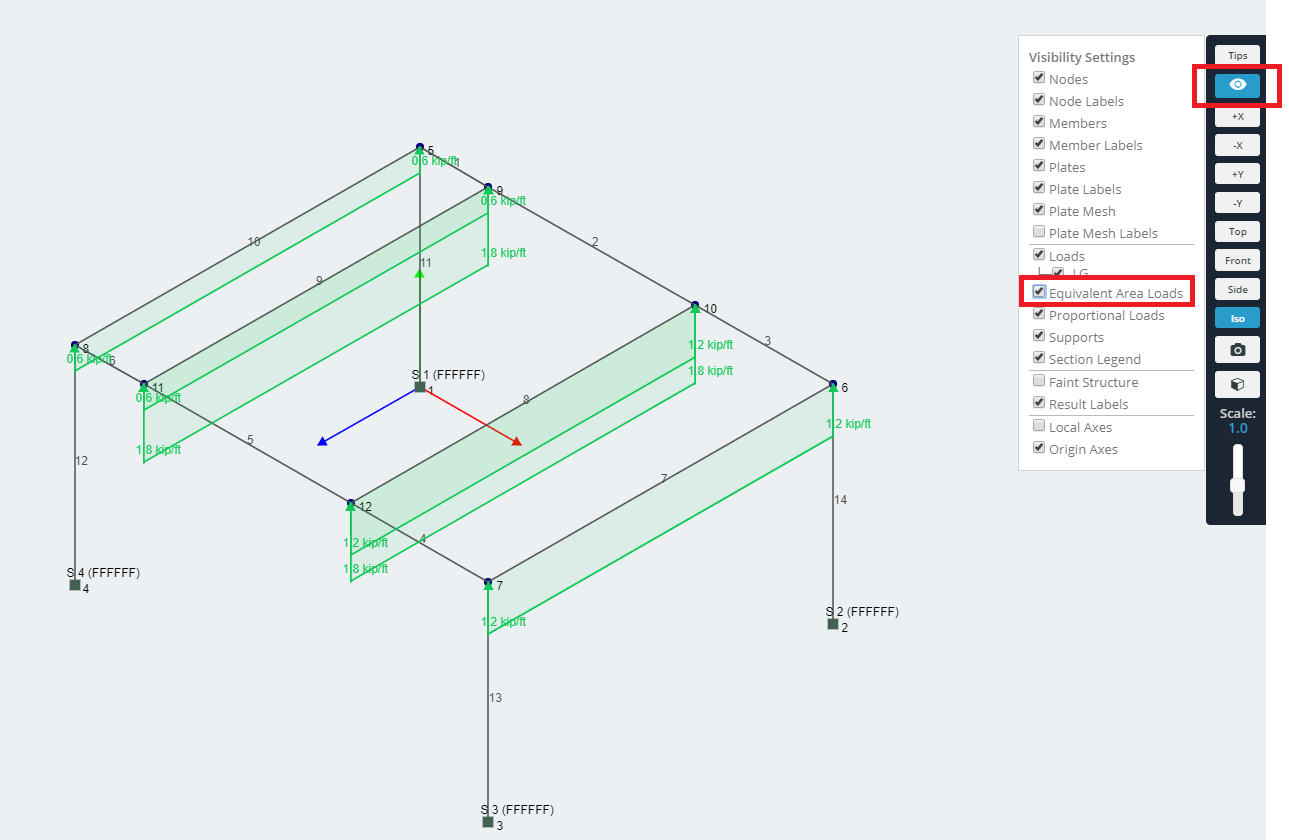Note the values presented here. The pressure is uniform, however the area loads are not. This can be expected since the spacing between the members will affect the result. As you can see, the spacing is highest between members 8 and 9, causing the area load to be highest, whilst the area load is lowest for member pair 9-10.

To change the value of the area load you simply go back to the menu and edit the value. Area loads are not directly converted to DL’s offering you the convenience to change the values at any time.

# Example: One Way (Closed) Area Load

This area load creates rectangular distributions of equal value across all of the members that it is applied to.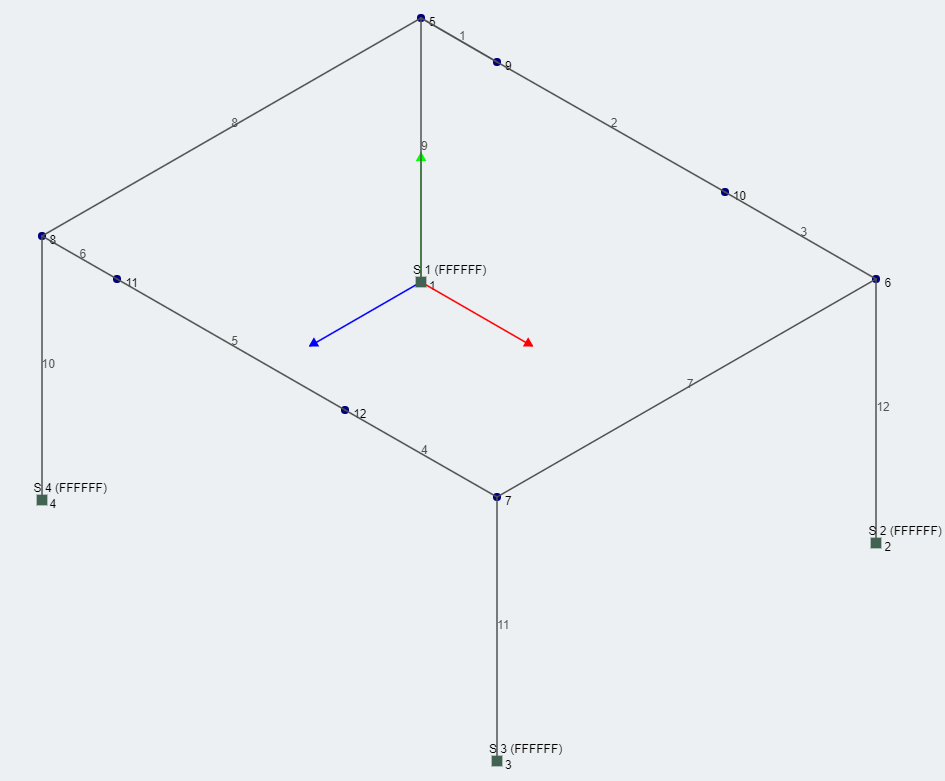Instead of specifying members, simply specify 3 or 4 nodes (for this example we'll specify the 4 outermost nodes), and the pressure value, and the area load will be generated.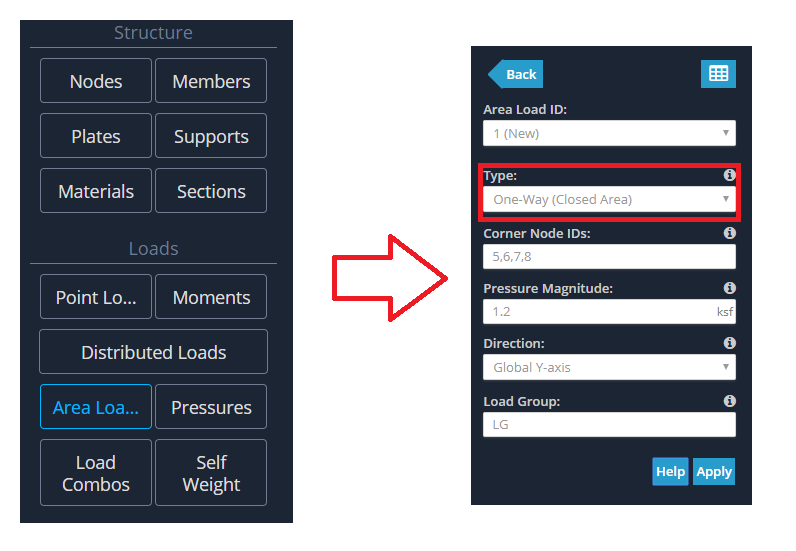Note that 8 members here have area loads applied to them, since they are all members that can be found when going from corner to corner along the plane.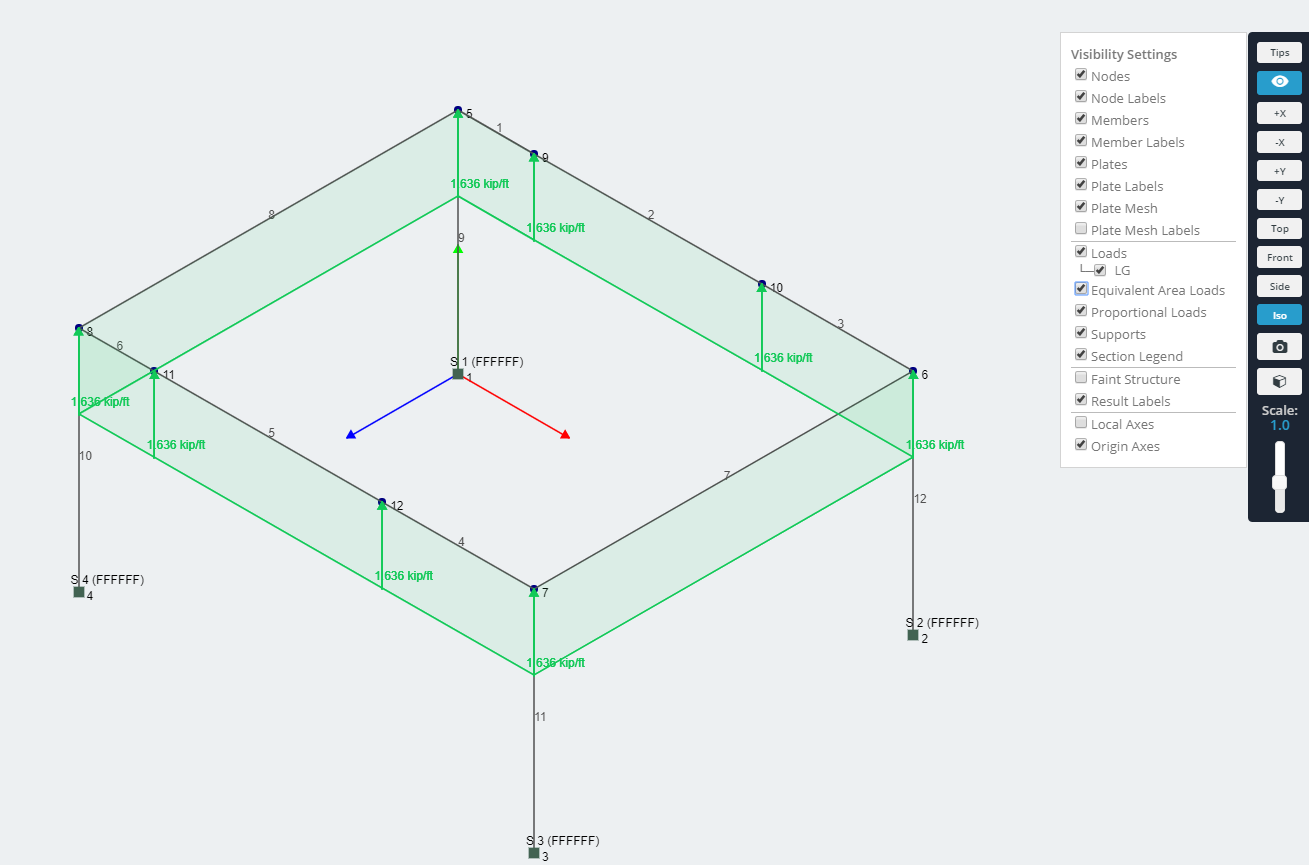# Example: Two Way Area Load

The next area load to consider is the two way area load. This assumes members in two directions support the pressure load from a slab, and the distribution of the distributed loads, is triangular on the shorter sides, and a quadrilateral on the other sides.

For this example, we'll be using the same frame geometry as previous.

To specifiy this you simply need to specify 3 or 4 nodes. For this example enter the 4 outermost nodes 5-8.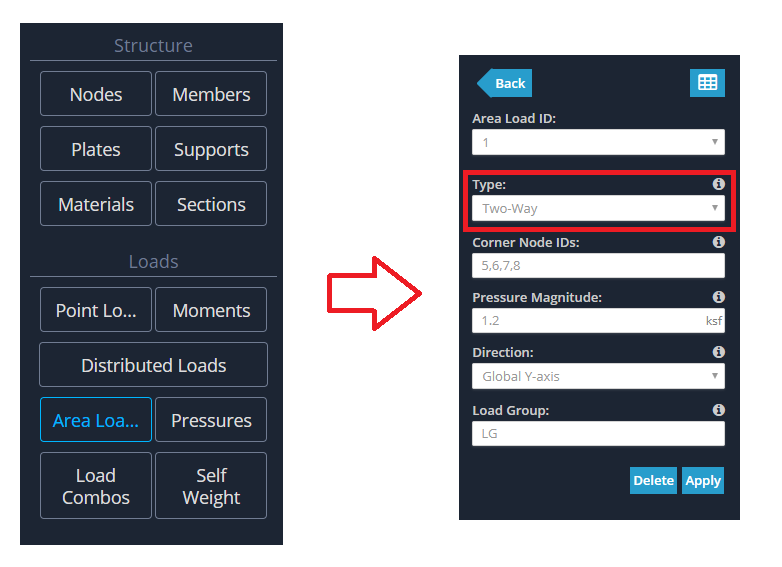This will automatically select the members that exist between corner to corner. The implementation is robust enough to detect multiple members that form the profile. For example, the side formed by nodes 6 and 7 will have a triangular DL applied to it. However there are 2 members between these two corner nodes. This doesn’t cause any problems at all, and the profile is generated perfectly.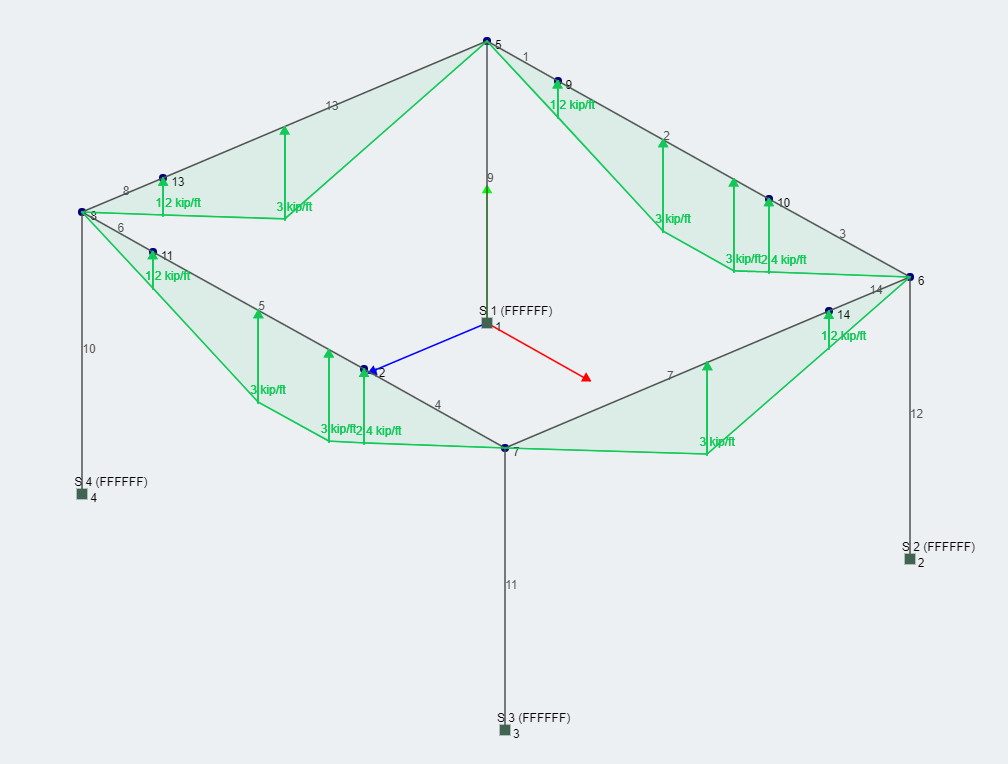The latest area load to be added is called the "Column Wind Load". It is different to the other area loads, because it is applied in the context of a wind load applied to a closed structure (like a wall resting on a set of columns). You are able to define a bounded plane without having that plane being bounded completely by members (overcoming the limitation of the previous area loads). You are also able to supply elevation points, and define pressures that are present in between those elevation points. As a general rule of thumb, you should have 1 less pressure value for the number of elevation points that you define.

Corner Nodes: List of three or four Node IDs which make up the corners/boundaries of the area load. The list of IDs needs to be seperated by commas (,) and in sequential order.

Elevations: Comma separated Elevations (Global Y Axis) for wind loads. These are the elevation ranges that match up to the Pressure Magnitudes below. For instance: 0,3,6 = 0-3m will coincide with the first Pressure Magnitu

Pressure Magnitudes: Comma separated Pressure Magnitudes for wind loads. These will be applied based on the above Elevations.

Column Direction: The direction of your columns. Enter only two node IDs separated by a comma e.g. 1,2.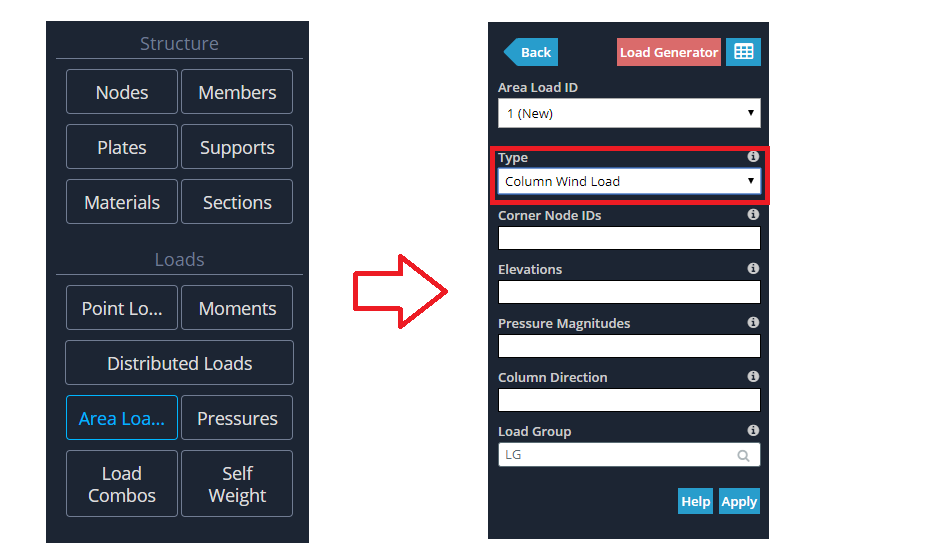You can offset from where your members start, and also stop before the members end. Note the columns are not connected by members at the base and that's ok.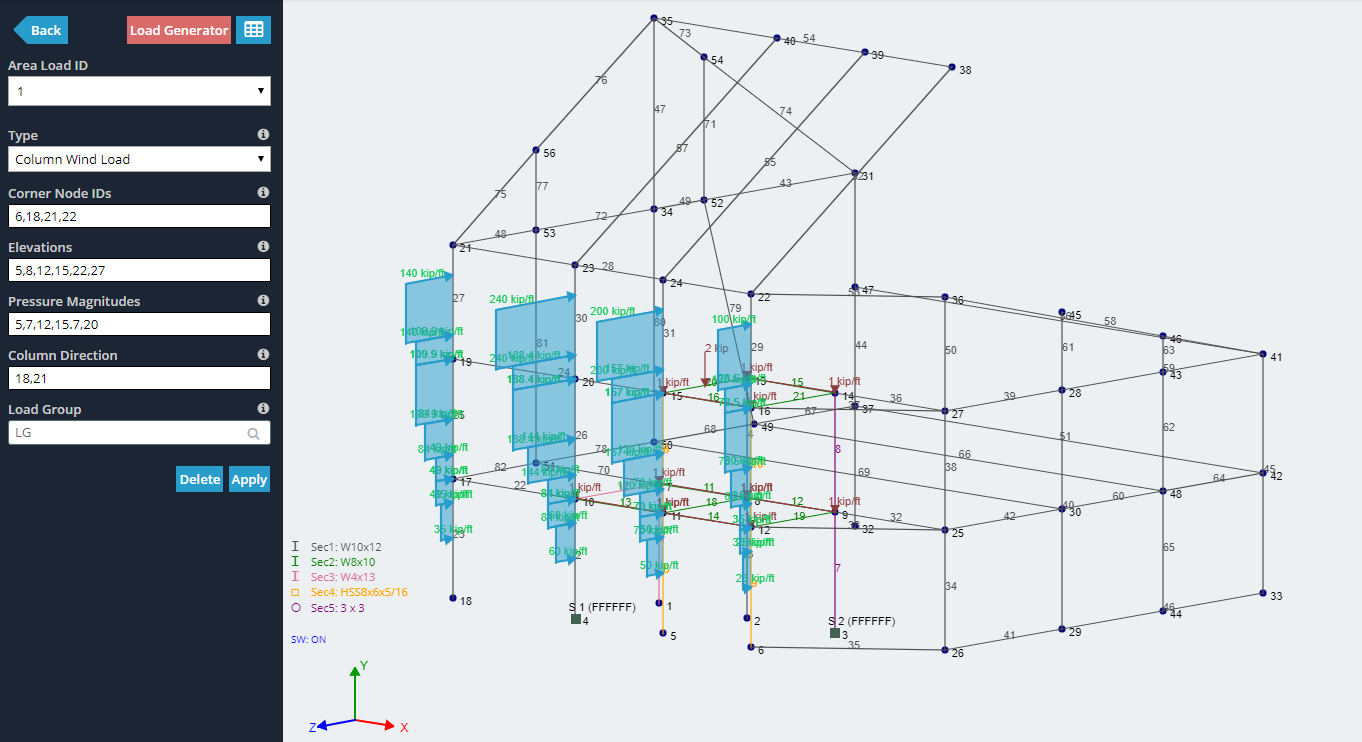You can set the direction of your column wind load with your defininition of the node order for the bounding plane. The direction is always perpendicular to your defined plane, however this gives 2 possibilities for direction. We follow the right hand rule for the direction here. Look at the example below, the corner node ID's is 6,22,21,18 (anti-clockwise) and if you curl your right hand in that direction, your thumb points in the direction of the generated wind load.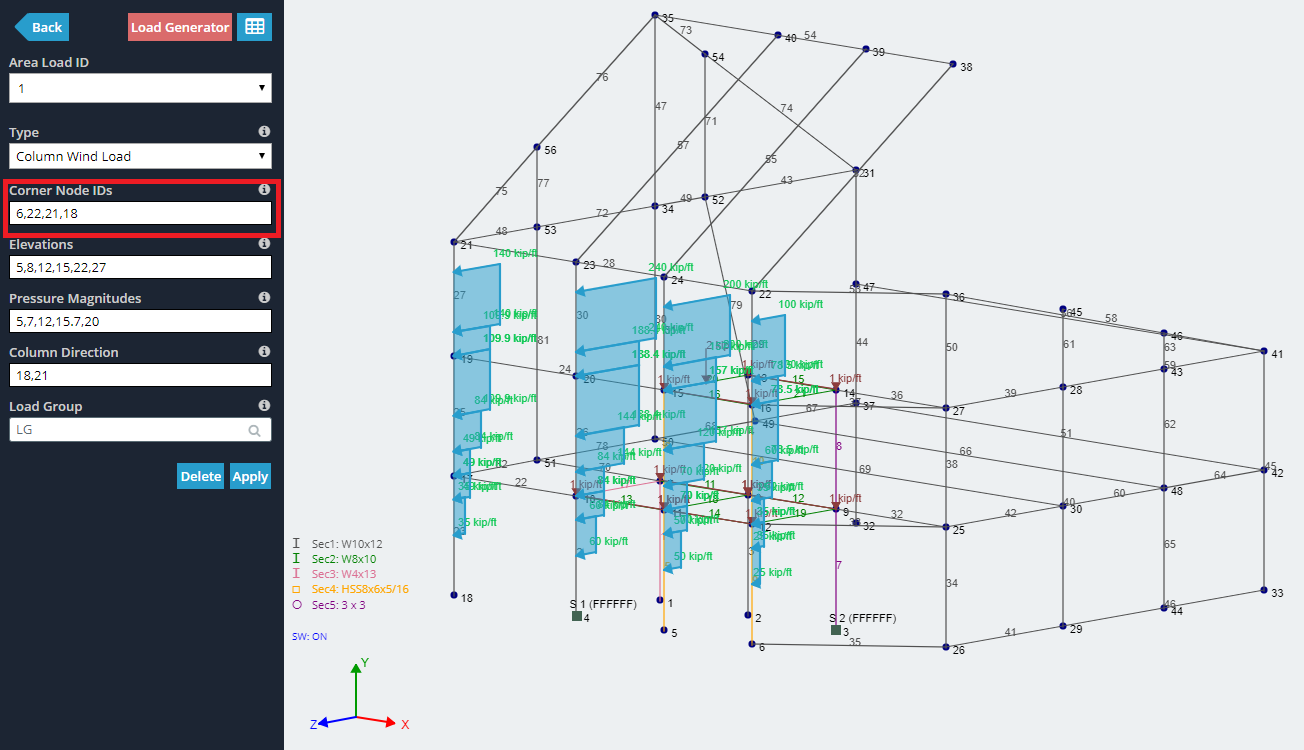You can also set a column wind load to a slanted roof.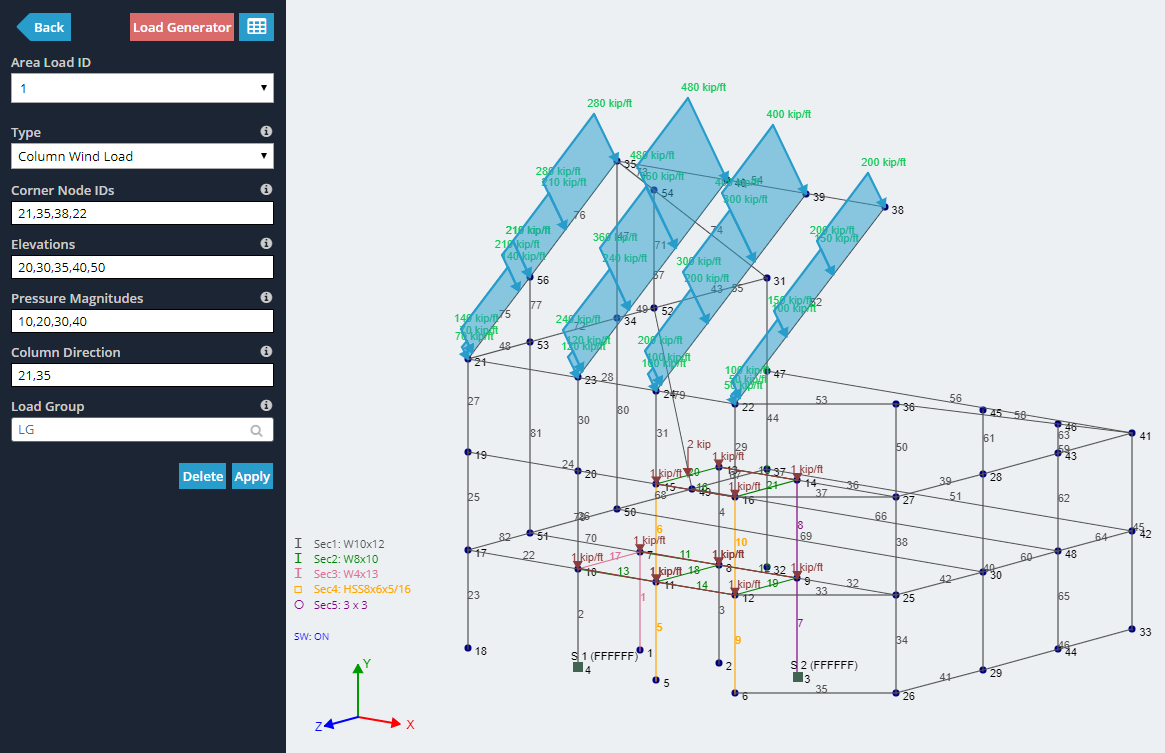You can also apply a wind load to a set of columns bounded by a triangular plane.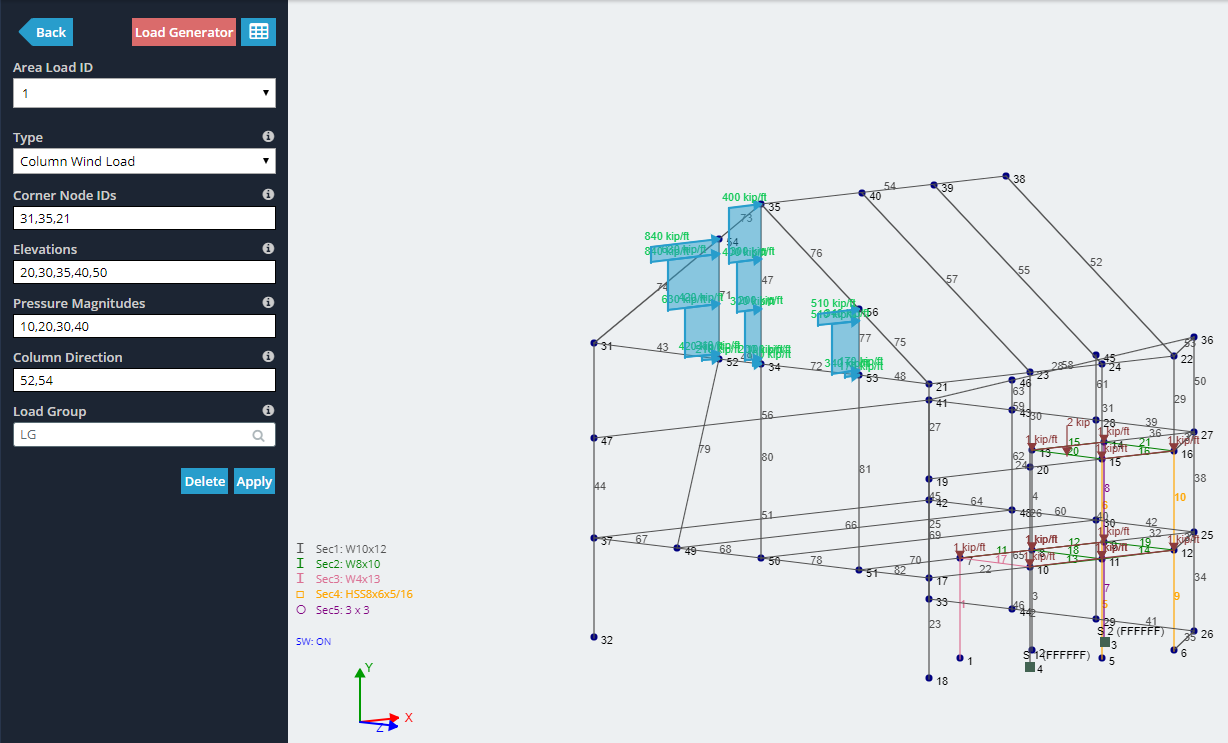If you're not able to see the column wind loads, you may need to change your view settings by checking the "Equivalent Area Loads" option.